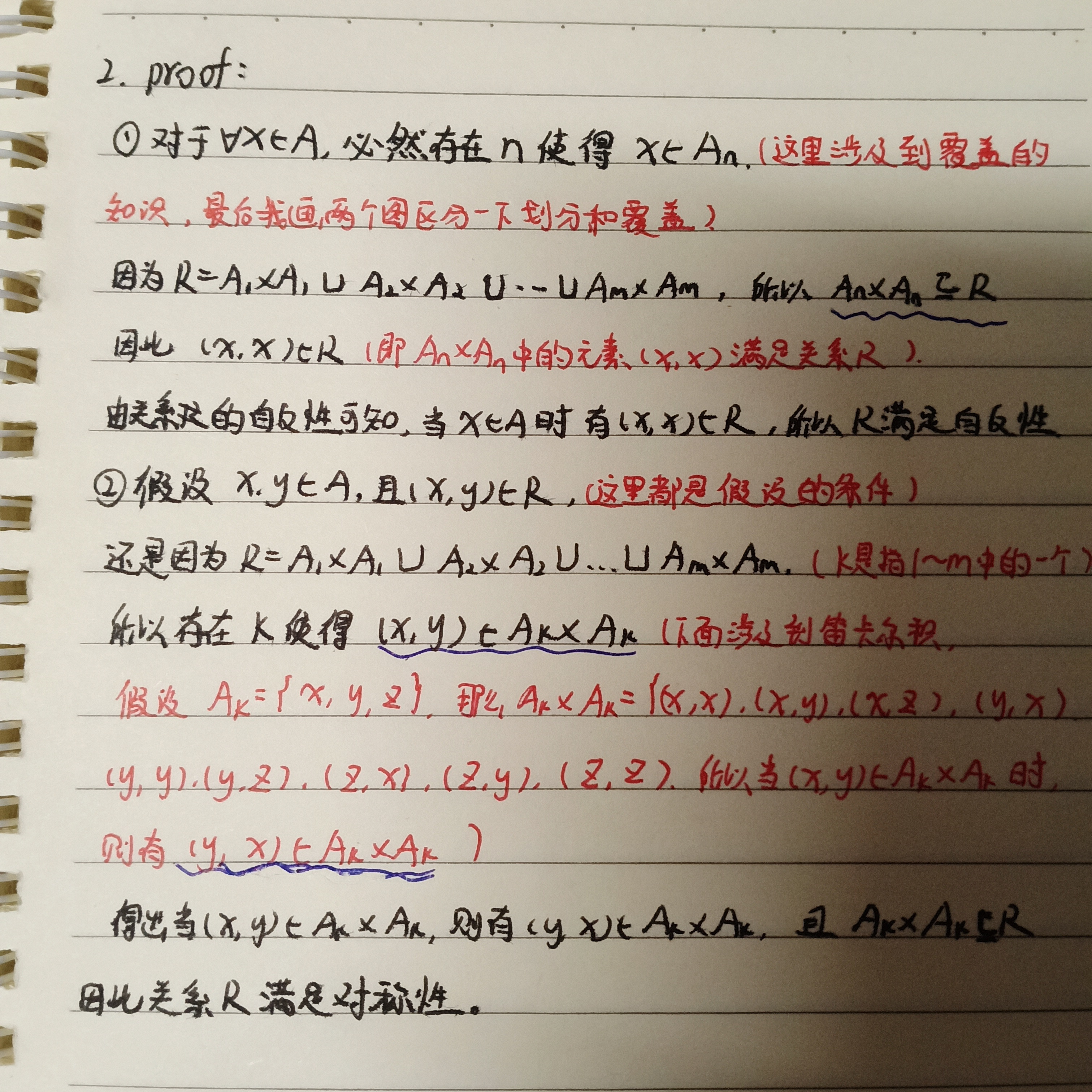# 集合、关系的性质、交换群与分配格的相关证明题

1.设A,B,C是非空有限集，证明：A-(B∪C)=(A-B)∩(A-C).
proof:
①设x∈A-(B∪C)，则有x∈A 且 x∉(B∪C)

②设x∈(A-B)∩(A-C)，则有x∈(A-B) 且 x∈(A-C)

2.给定集合A的覆盖{A₁，A₂，A₃,…Aₘ}，证明由它确定的关系R=A₁×A₁∪A₂×A₂∪…∪Aₘ×Aₘ是相容关系.（即满足自反和对称的二元关系）
proof：
①对于∀x∈A，显然∃n使得x∈Aₙ，所以(x,x)∈R，故R是自反
②若x,y∈A，且(x,y)∈R，则必∃k使得(x,y)∈Aₖ×Aₖ，所以(y,x)∈Aₖ×Aₖ，3.在整数集合Z上定义○运算，如x,y∈Z，x○y=x+y-2.

proof：
①在整数集合Z上处处有定义，输出值唯一且x○y=x+y-2∈Z(封闭性)，所以○运算是二元运算
②(x○y)○z=(x+y-2)○z=(x+y-2+z)-2=x+y+z-4
x○(y○z)=x○(y+z-2)=(x+y+z-2)-2=x+y+z-4

(到这里已经可以证明(Z,○)是半群啦)
③∃2∈Z，使得2○x=x○2=x，所以(Z,○)是有幺元identity的半群
(说明2为幺元呀,到这里可以说明它是个幺元半群了，也就是拟群monoid)
④对∀x∈Z，都有4-x使得x○(4-x)=(4-x)○x=2，所以(Z,○)有逆元inverse，也就是说(Z,○)是一个群
⑤对∀x,y∈Z,x○y=x+y-2，y○x=y+x-2，得出x○y=y○x，所以(Z,○)满足交换律

(相关知识在《离散数学结构》362页)
4.令(L,≤)是一个分配格(distributive lattice)，证明:如果存在a∈L，使得a∧x=a∧y，并且a∨x=a∨y，那么就有x=y.
proof:
Suppose a∧x=a∧y and a∨x=a∨y.
Then y≤y∨(y∧a)=(y∧y)∨(y∧a)
=y∧(y∨a)
=y∧(a∨x)
=(y∧a)∨(y∧x)
=(a∧x)∨(y∧x)
=x∧(a∨y)≤x
Hence y≤x.
Similarly, x≤x∨(x∧a)=(x∧x)∨(x∧a)
=x∧(x∨a)
=x∧(a∨y)
=(x∧a)∨(x∧y)
=(a∧y)∨(x∧y)
=y∧(a∨x)≤y
Hence x≤y.
Thus x=y.

a∧(b∨c)=(a∧b)∨(a∧c)
a∨(b∧c)=(a∨b)∧(a∨c)
(《离散数学结构》242页练习题25)

07-18329703-131万+
04-166621
06-031万+
03-181万+
04-053540
01-081897
03-152699
09-301576
06-203万+
03-014150
07-182万+
04-193732
05-176540
03-104万+
09-296036
12-0621万+
09-231万+
01-239711
05-292万+